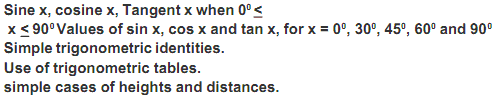NEW!  CBSE Papers PDF: Class-X, Class-XII

# Combined Defence Services Examination (CDS) - Syllabus

Disclaimer: This website is not at associated with CBSE, For official website of CBSE visit - www.cbse.nic.in

## SCHEME OF EXAMINATION:

1. The Competitive examination comprises:
(a) Written examination as shown in para 2 below.
(b) Interview for intelligence and personality test (vide Part ‘B’ of this Appendix) of such candidates as may be called for interview at one of the Services Selection Centres.

2. The subjects of the written examination, the time allowed and the maximum marks allotted to each subject will be as follows:

English
• Time Duration: 2 hrs.
• Maximum Marks: 100

General Knowledge

• Time Duration: 2 hrs.
• Maximum Marks: 100

Elementary Mathematics

• Time Duration: 2 hrs.
• Maximum Marks: 100

English
• Time Duration: 2 hrs.
• Maximum Marks: 100

General Knowledge

• Time Duration: 2 hrs.
• Maximum Marks: 100

The maximum marks allotted to the written examination and to the interviews will be equal for each course i.e. the maximum marks allotted to the written examination and to the interviews will be 300, 300, 300 and 200 each for admission to the Indian Military Academy, Indian Naval Academy, Air Force Academy and Officers’ Training Academy respectively.

3. The papers in all the subjects will consist of objective type questions only. The question papers (Test Booklets) of General Knowledge and Elementary Mathematics will be set bilingually in Hindi as well as English.

4. In the question papers, wherever necessary, questions involving the metric system of Weights and Measures only will be set.

5. Candidates must write the papers in their own hand. In no circumstances will they be allowed the help of a scribe to write answers for them.

6. The Commission have discretion to fix qualifying marks in any or all the subjects of the examination.

7. The candidates are not permitted to use calculator for answering objective type papers (Test Booklets). They should not therefore, bring the same inside the Examination Hall.

## CDS (I) Examination Syllabus:

STANDARD: The standard of the papers in Elementary Mathematics will be of Matriculation level. The standard of papers in other subjects will approximately be such as may be expected of a graduate of an Indian University.

ENGLISH (CODE No. 01)

• The question paper will be designed to test the candidates’ understanding of English and workmanlike use of words.

GENERAL KNOWLEDGE (Code No. 02)

• General Knowledge including knowledge of current events and of such matters of everyday observation and experience in their scientific aspects as may be expected of an educated person who has not made a special study of any scientific subject. The paper will also include questions on History of India and Geography of a nature which candidate should be able to answer without special study.

ELEMENTARY MATHEMATICS (Code No. 03)

• ARITHMETIC: Number System-Natural numbers, Integers, Rational and Real numbers. Fundamental operations addition, subtraction, multiplication, division, Square roots, Decimal, fractions. Unitary method, time and distance, time and work, percentages, applications to simple and compound interest, profit and loss, ratio and proportion, variation. Elementary Number Theory- Division algorithm. Prime and composite numbers. Tests of divisibility by 2,3,4,5,9 and 11. Multiples and factors. Factorisation Theorem. H.C.F. and L.C.M. Euclidean algorithm, Logarithms to base 10, laws of logarithms, use of logarithmic tables.

• ALGEBRA: Basic Operations, simple factors, Remainder Theorem, H.C.F., L.C.M. Theory of polynomials, solutions of quadratic equations, relation between its roots and coefficients (Only real roots to be considered). Simultaneous linear equations in two unknowns-analytical and graphical solutions. Simultaneous linear inequations in two variables and their solutions. Practical problems leading to two simultaneous linear equations or inequations in two variables or quadratic equations in one variable & their solutions. Set language and set notation, Rational expressions and conditional identities, Laws of indices.

• TRIGONOMETRY:• GEOMETRY: Lines and angles, Plane and plane figures, Theorems on (i) Properties of angles at a point (ii) Parallel lines, (iii) Sides and angles of a triangle, (iv) Congruency of triangles, (v) Similar triangles, (vi) Concurrence of medians and altitudes, (vii) Properties of angles, sides and diagonals of a parallelogram, rectangle and square (viii) Circles and its properties including tangents and normals, (ix) Loci.

• MENSURATION: Areas of squares, rectangles, parallelograms, triangle and circle. Areas of figures which can be split up into these figures (Field Book), Surface area and volume of cuboids, lateral surface and volume of right circular cones and cylinders, surface area and volume of spheres.

• STATISTICS: Collection and tabulation of statistical data, Graphical representation, frequency polygons, histograms, bar charts, pie charts etc. Measures of central tendency.

INTELLIGENCE AND PERSONALITY TEST:

• In addition to the interview the candidates will be put to Intelligence Tests both verbal and non-verbal, designed to assess their basic intelligence. They will also be put to Group Tests such as group discussions, group planning, outdoor group tasks, and asked to give brief lectures on specified subjects. All these tests are intended to judge the mental calibre of a candidate. In broad terms, this is really an assessment of not only his/her intellectual qualities but also his/her social traits and interests in current affairs.

»»

NEW!  CBSE Papers PDF: Class-X, Class-XII